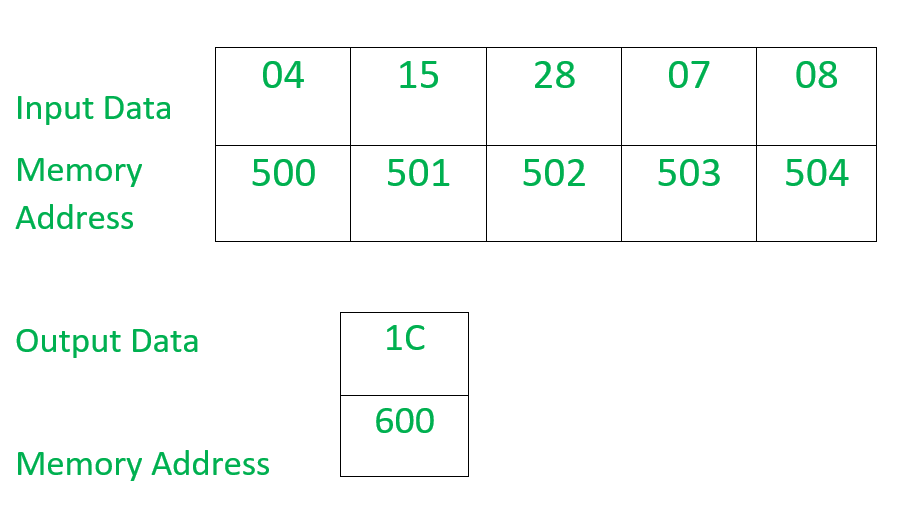Open in App
Not now

# 8086 program to find sum of odd numbers in a given series

• Last Updated : 01 Jun, 2021

Problem – Write an Assembly Language Program to find sum of odd numbers in a given series containing 8 bit numbers stored in a continuous memory location and store the result in another memory location.

Example –Example Explanation –

1. 500 offset stores the counter value of the series and the elements of the series starts from 501 to 504 offset.
2. In this example, we have 4 terms only. Adding the odd terms (found in BL register) 15+07 in AL register and result gets stored (1C) in AL register.
3. The result from AL register gets stored in 600 offset.

Assumptions –

1. The counter value which tells that how many numbers are there in the series is stored at memory location 500.
2. The elements of the series are stored in a continuous memory location starting from 501.
3. The result is stored at a memory location 600.
4. The starting address of the program is 400.

Test –

`Syntax: TEST d, s `

It performs AND operation of destination(d) and source(s) but result is not stored only flags ate modified.

Algorithm –

1. Load data from offset 500 to register CL.
2. Increment the value of offset.
3. Load 00H into CH register.
4. Load 00H into AL register.
5. Load data from offset to register BL.
6. Use TEST instruction to check whether data in BL is even or odd, if zero flag is set means data is even then go to step 7 otherwise data is odd then go to step 8.
8. Add the data of AL and BL registers and store the result in AL register.
9. Increment the value of offset.
10. Jump to memory location 40AH if content of CX is not equal to zero otherwise go to step 11.
11. Load the data from AL register to memory location 600.
12. End

Program –

Explanation –

1. MOV SI, 500 load the value 500 to SI.
2. MOV CL, [SI] loads the data of offset SI into CL register.
3. INC SI increases the value of SI by one.
4. MOV CH, 00 loads the value 00 into CH register.
5. MOV AL, 00 loads the value 00 into AL register.
6. MOV BL, [SI] loads the data of offset SI into BL register.
7. TEST BL, 01 AND operation of content of BL and value 01 and flag registers are modified.
8. JZ 413 jump to 413 memory location if zero flag is set.
9. ADD AL, BL add the contents of AL and BL registers and store the result in AL register.
10. INC SI increases the value of SI by one.
11. LOOP 40A jump to 40A memory location if value of CX is not equal to zero.
12. MOV , AL loads the content of AL register to memory location 600.
13. HLT stops the execution of the program.
My Personal Notes arrow_drop_up